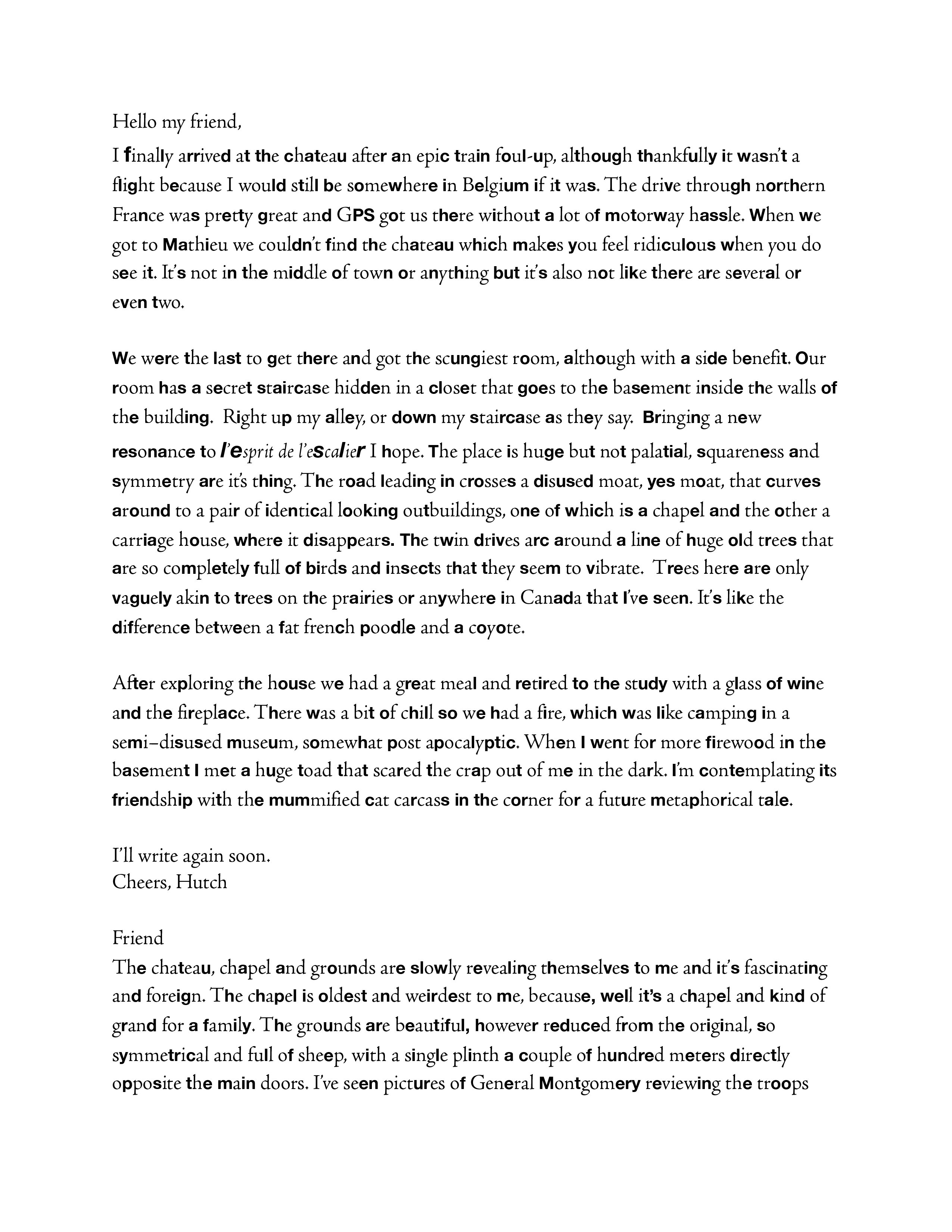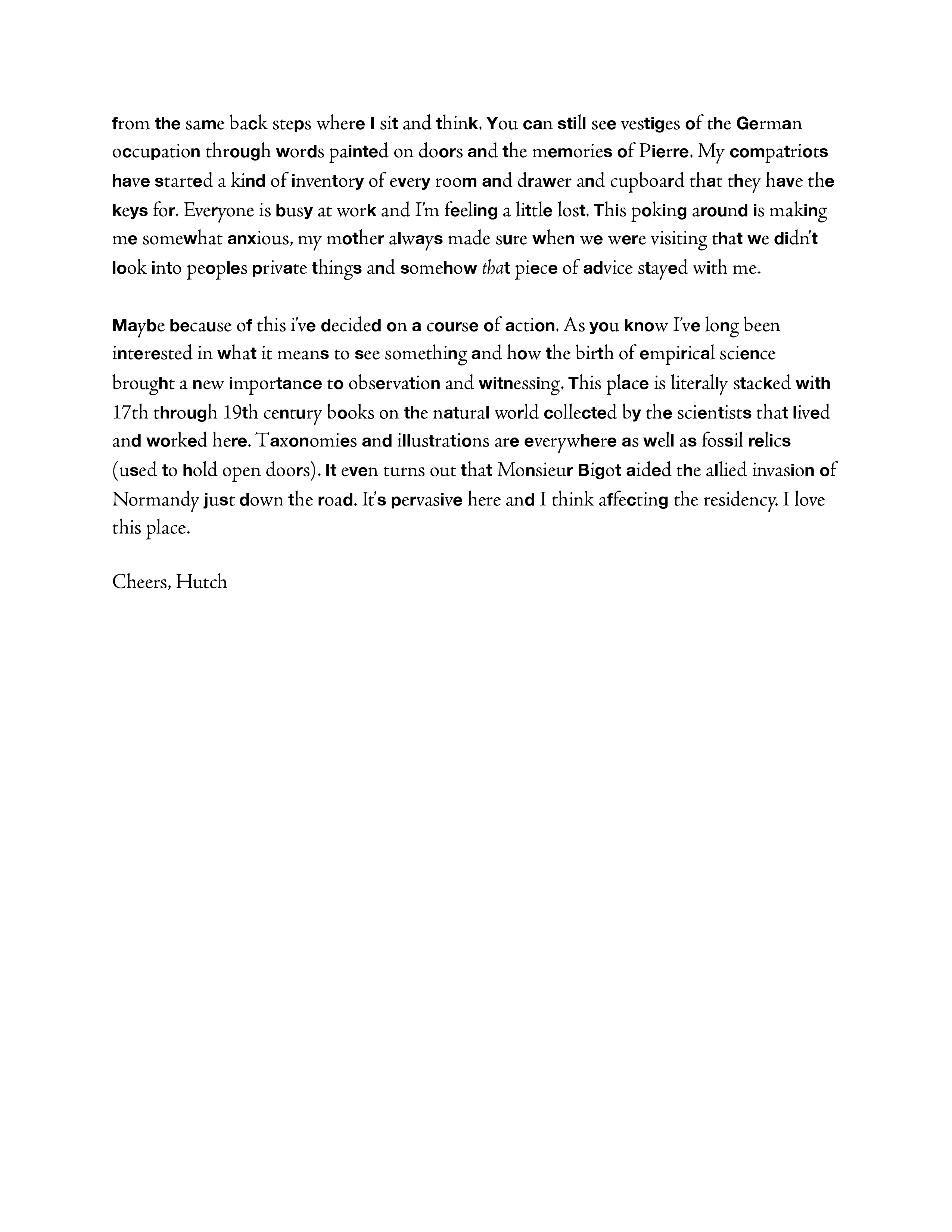## Chateau Mathieu

A residency in Normandy in 2009

## The Accompanying Text in a cipher form

Baconian Cipher

The Baconian Ciphers are based on a binary encoding of the plain text.  Each letter is assigned to a string of five binary digits.  In most cases, a and b are used instead of 1 and 0.  A simple Baconian Cipher Encoding might be:

A = aaaaa  I/J = abaaa    R = baaaa

B = aaaab    K = abaab    S = baaab

C = aaaba    L = ababa    T = baaba

D = aaabb    M = ababb  U/V = baabb

E = aabaa    N = abbaa    W = babaa

F = aabab    O = abbab    X = babab

G = aabba    P = abbba    Y = babba

H = aabbb    Q = abbbb    Z = babbb

Where is a serif font and b is sans serif

The salutation and signature lines are not included in the cipher